# How to Calculate and Solve for Knudsen Diffusion of Moulding Sand | Mass Transfer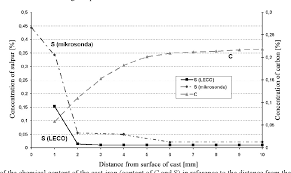The image above represents knudsen diffusion of moulding sand.

To compute for knudsen diffusion of moulding sand, three essential parameters are needed and these parameters are Pore Radius (r), Temperature (T) and Molecular Weight (M).

The formula for calculating knudsen diffusion of moulding sand:

Dk = 9700r√(T/M)

Where:

Dk = Knudsen Diffusion of Moulding Sand
T = Temperature
M = Molecular Weight

Let’s solve an example;
Find the knudsen diffusion of moulding sand when the pore radius is 24, the temperature is 18 and the molecular weight is 12.

This implies that;

r = Pore Radius = 24
T = Temperature = 18
M = Molecular Weight = 12

Dk = 9700r√(T/M)
Dk = 9700(24)√(18/12)
Dk = 9700(24)√(1.5)
Dk = 9700(24)(1.22)
Dk = 285120.6

Therefore, the knudsen diffusion of moulding sand is 285120.6 cm²/s.

Calculating the Pore Radius when the Knudsen Diffusion of Moulding Sand, the Temperature and the Molecular Weight is Given.

r = Dk / 9700 √(T / M)

Where:

Dk = Knudsen Diffusion of Moulding Sand
T = Temperature
M = Molecular Weight

Let’s solve an example;
Find the pore radius when the knudsen diffusion of moulding sand is 20, the temperature is 14 and the molecular weight is 10.

This implies that;

Dk = Knudsen Diffusion of Moulding Sand = 20
T = Temperature = 14
M = Molecular Weight = 10

r = Dk / 9700 √(T / M)
r = 20 / 9700 √(14 / 10)
r = 20 / 9700 √(1.4)
r = 20 / 11446
r = 0.00174

Therefore, the pore radius is 0.00174.

Calculating the Temperature when the Knudsen Diffusion of Moulding Sand, the Pore Radius and the Molecular Weight is Given.

T = M x (Dk / 9700r)2

Where:

T = Temperature
Dk = Knudsen Diffusion of Moulding Sand
M = Molecular Weight

Let’s solve an example;
Find the temperature when the knudsen diffusion of moulding sand is 2700, the pore radius is 6 and the molecular weight is 14.

This implies that;

Dk = Knudsen Diffusion of Moulding Sand = 2700
r = Pore Radius = 6
M = Molecular Weight = 14

T = M x (Dk / 9700r)2
T = 14 x (2700 / 9700(6))2
T = 14 x (2700 / 58200)2
T = 14 x (0.046)2
T = 14 x 0.002116
T = 0.0296

Therefore, the temperature is 0.0296 s.

Calculating the Molecular Weight when the Knudsen Diffusion of Moulding Sand, the Temperature and the Pore Radius is Given.

M = T / (Dk / 9700r)2

Where:

M = Molecular Weight
Dk = Knudsen Diffusion of Moulding Sand
T = Temperature

Let’s solve an example;
Find the molecular weight when the knudsen diffusion of moulding sand is 3000, the pore radius is 8 and the temperature is 4.

This implies that;

Dk = Knudsen Diffusion of Moulding Sand = 3000
r = Pore Radius = 8
T = Temperature = 4

M = T / (Dk / 9700r)2
M = 4 / (3000 / 9700(8))2
M = 4 / (3000 / 77600)2
M = 4 / 0.00148
M = 2702.7

Therefore, the molecular weight is 2702.7 cm.

Nickzom Calculator – The Calculator Encyclopedia is capable of calculating the knudsen diffusion of moulding sand.

To get the answer and workings of the knudsen diffusion of moulding sand using the Nickzom Calculator – The Calculator Encyclopedia. First, you need to obtain the app.

You can get this app via any of these means:

To get access to the professional version via web, you need to register and subscribe for NGN 2,000 per annum to have utter access to all functionalities.
You can also try the demo version via https://www.nickzom.org/calculator

Apple (Paid) – https://itunes.apple.com/us/app/nickzom-calculator/id1331162702?mt=8
Once, you have obtained the calculator encyclopedia app, proceed to the Calculator Map, then click on Materials and Metallurgical under Engineering.Now, Click on Mass Transport under Materials and MetallurgicalNow, Click on Knudsen Diffusion of Moulding Sand under Mass Transport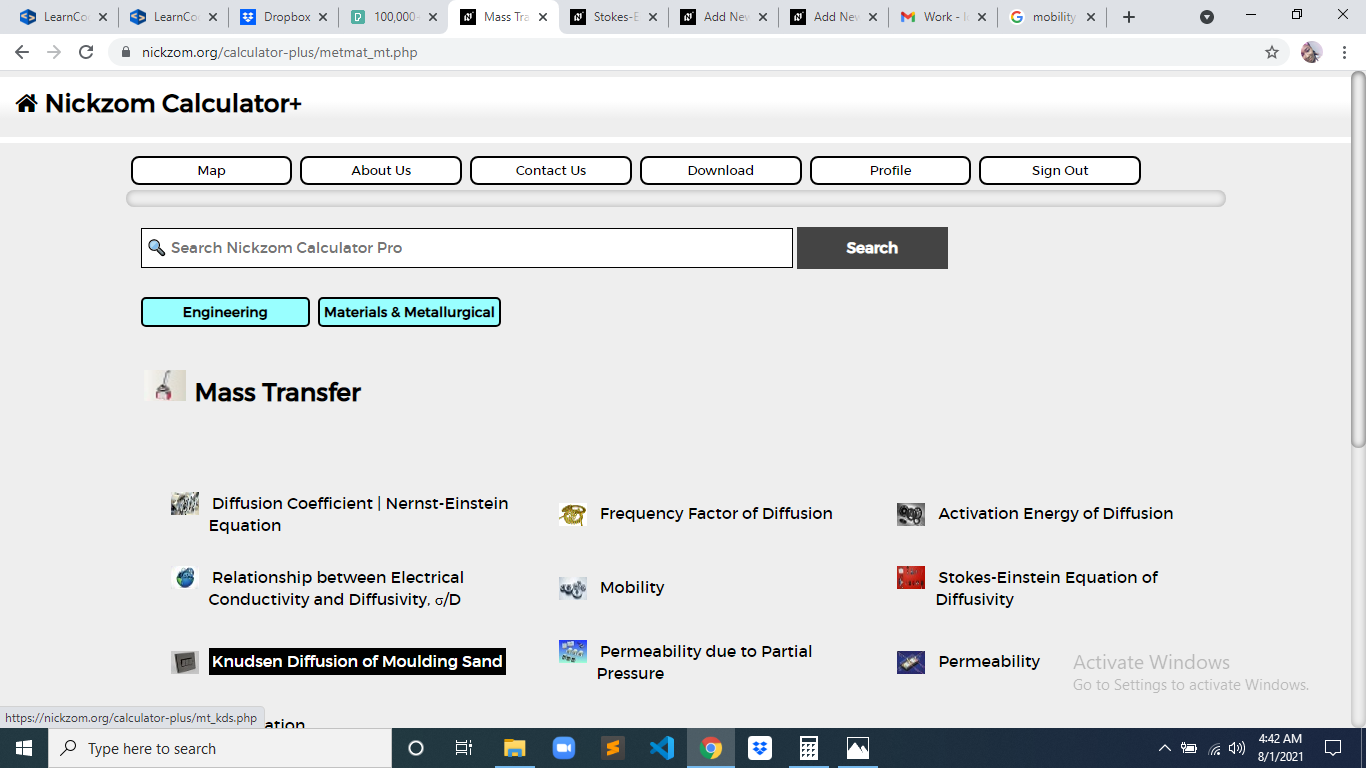The screenshot below displays the page or activity to enter your values, to get the answer for the knudsen diffusion of moulding sand according to the respective parameter which is the Pore Radius (r), Temperature (T) and Molecular Weight (M).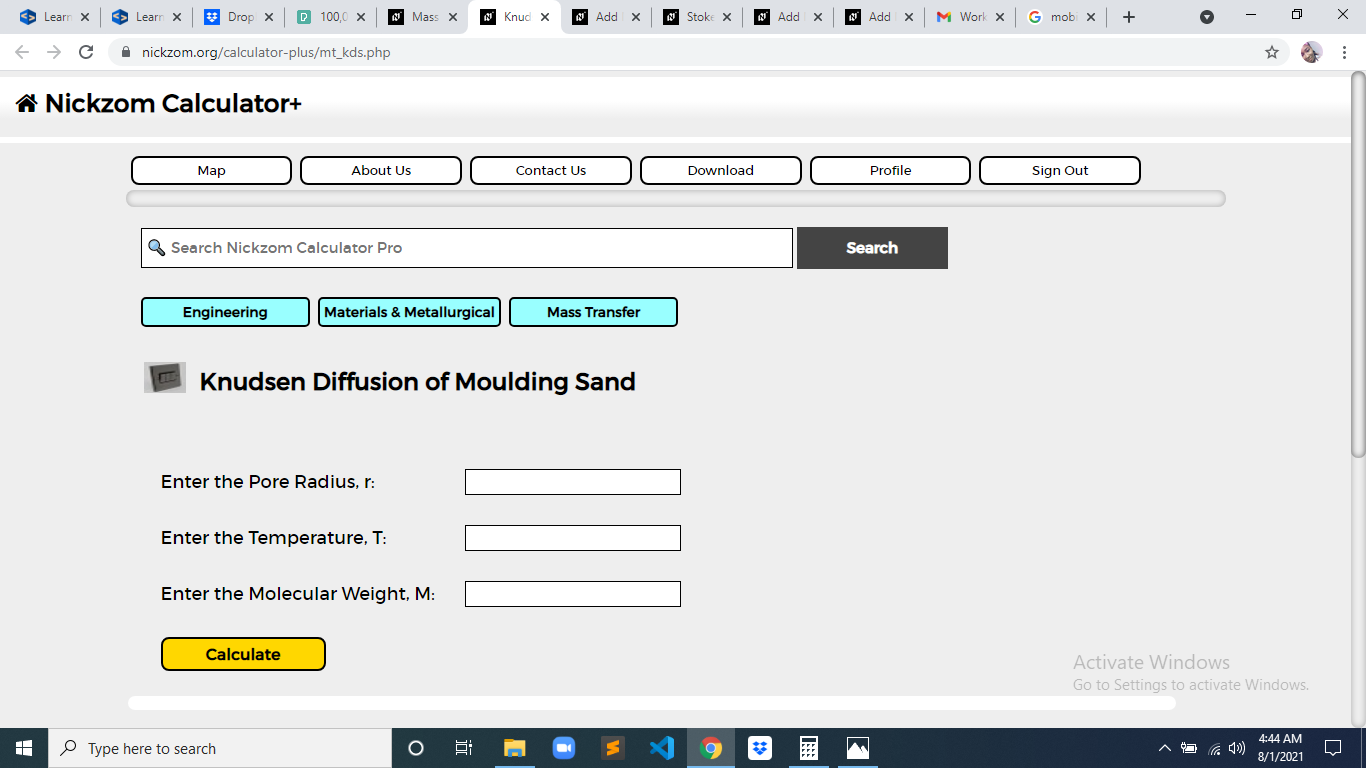Now, enter the values appropriately and accordingly for the parameters as required by the Pore Radius (r) is 24, Temperature (T) is 18 and Molecular Weight (M) is 12.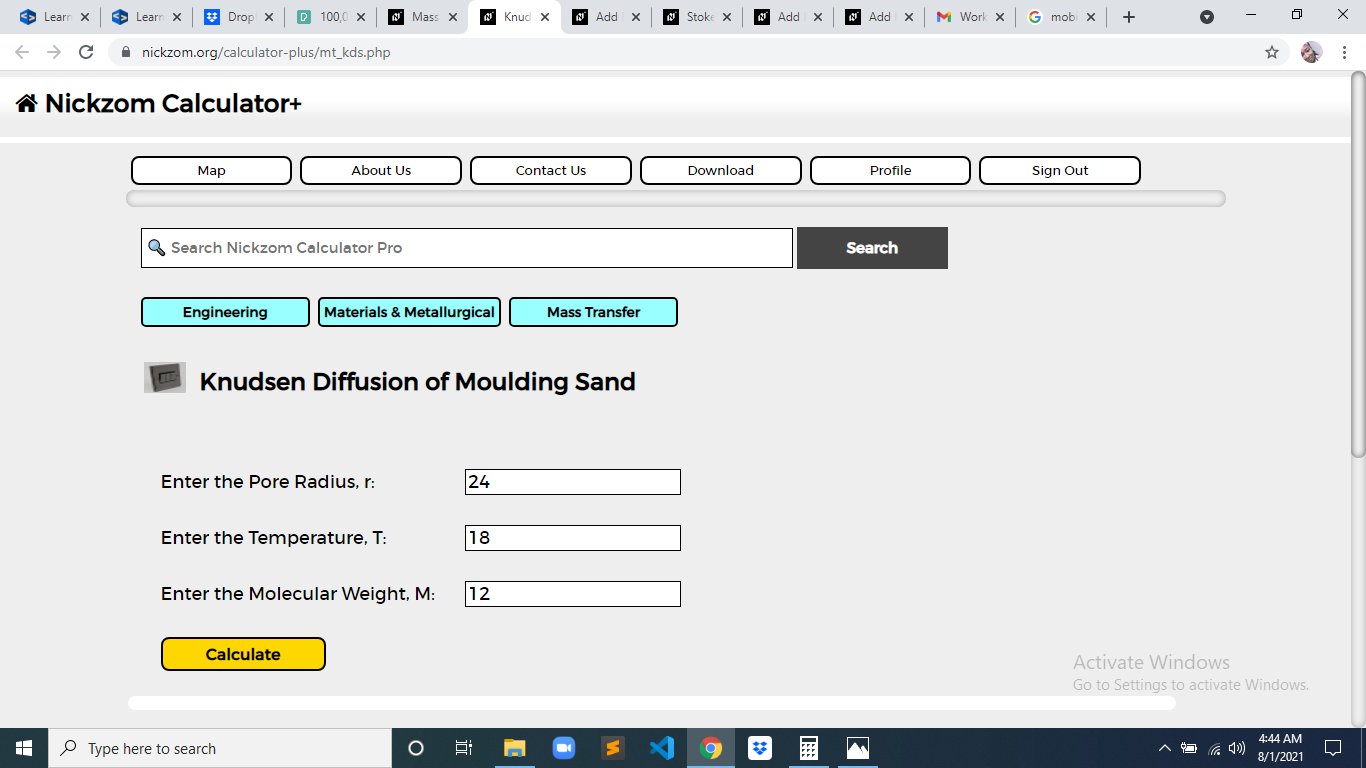Finally, Click on Calculate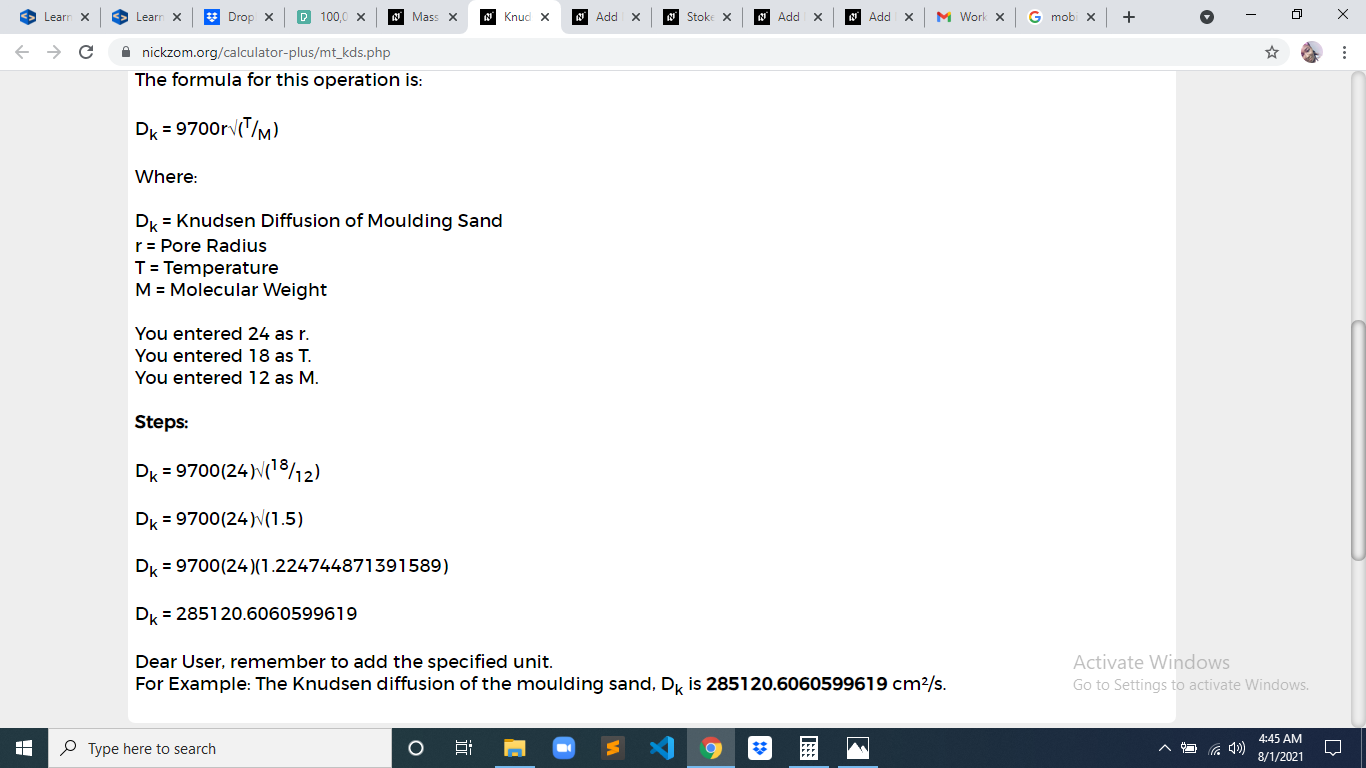As you can see from the screenshot above, Nickzom Calculator– The Calculator Encyclopedia solves for the knudsen diffusion of moulding sand and presents the formula, workings and steps too.